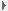Home About Journal Editorial Board Instruction Subscriptions Contacts Us 中文2008, Vol. 40Issue (01) : 101-108     DOI:|Methodology of Equating Based on Probability Distribution and Its Applications
DING Shu-Liang;WU Rui;ZHANG Jie-Lan;XIONG Jian-Hua
Computer Information Engineer College, JiangXi Normal University, Nanchang, Jiangxi 330027, China
 Download: PDF(1032 KB)   Export: BibTeX | EndNote | Reference Manager | ProCite | RefWorks
Abstract  This paper, divided into two parts, discusses the following two issues: (1) the methodology of developing a new test equating criterion and (2) the behavior of a new test equating method, referred to as cosine criterion.
Under the item response theory (IRT) and in light of the probability distribution of an examinee’s response to some item, the first part of this paper proposes the methodology derived from the published literature on some test equating criteria. Moreover, some test equating criteria could be regarded as certain functions of probability distributions. Based on this, a series of test equating approaches, such as the Haebara item characteristic curve equating method (Hcrit), Stocking-Lord test characteristic curve equating method (SLcrit), logcontract equating method, SQRT method, and weighted Haebara method, could be clearly illustrated. Further, the relationship between Hcrit and SLcrit was identified: if the mutual compensation of the responses to the anchor items is evident, then SLcrit is suitable, and if not, then Hcrit is more appropriate.
In the second part of the paper, a new test equating criterion, known as cosine criterion (COScrit) was discussed as an example of the application of this methodology of the equating criteria. The results of the Monte Carlo study show that the behavior of the new criterion is better than that of Hcrit and SLcrit; this is evident when the data is fit to the generalized partial credit model (GPCM) in the sense that the root mean squared deviations (RMSDs) corresponding to the three criteria are compared. Further, the RMSD to COScrit is smaller and statistically significant. When the data is fit to the 2-parameter logistic model 2PLM, or the graded response model (GRM), COScrit is comparable to SLcrit; in fact, it is considerably better than SLcrit, provided that the equating coefficient A is not smaller than 1.2. If, however, coefficient A is smaller than 1.2, an inverse result is observed. Nevertheless, COScrit is inferior to Hcrit in both the cases. The findings suggest that the behavior of a test equating criterion is related to the domain of coefficient A, particularly to the item response function (IRF) .
 : B841
Corresponding Authors: DING Shu-Liang
Issue Date: 30 January 2008
 ServiceE-mail this articleE-mail AlertRSS Articles by authors
 Cite this article: DING Shu-Liang,WU Rui,ZHANG Jie-Lan,XIONG Jian-Hua. Methodology of Equating Based on Probability Distribution and Its Applications[J]. ,2008, 40(01): 101-108. URL: http://journal.psych.ac.cn/xlxb/EN/     OR     http://journal.psych.ac.cn/xlxb/EN/Y2008/V40/I01/101
  WANG Wenyi; DING Shuliang; SONG Lihong. Distance Discrimination Method based on Conditional Expectation in Cognitive Diagnosis[J]. Acta Psychologica Sinica, 2015, 47(12): 1499-1510.  WU Rui,DING Shu-Liang,GAN Deng-Wen. Test Equating with Testlets[J]. , 2010, 42(03): 434-442.  Ding Shuliang, Xiong Jianhua, Luo Fen, Wu Rui, Gan Xiaofang, Tu Bai. A NEW EQUATING CRITERION AND ITS BEHAVIORS[J]. , 2005, 37(05): 674-680.
Viewed
Full text

Abstract

Cited

Shared
Discussed